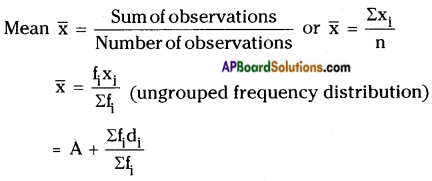Students can go through AP Board 9th Class Maths Notes Chapter 9 Statistics to understand and remember the concepts easily.

## AP State Board Syllabus 9th Class Maths Notes Chapter 9 Statistics

→ The facts or figures which are numerical or otherwise collected with a definite purpose are called data.

→ Statistics is the branch of mathematics which studies about the data and its meaning.

→ The information collected by the investigator with a definite objective is called primary data.

→ The information collected from a source, which had already been recorded lay from registers is called secondary data.

→ Data exists in raw form.→ Data is classified into groups to make the study easy.

→ The difference between the minimum and maximum values of a data is called the range of the given data.

→ The table showing the actual observations with their frequencies is called ungrouped frequency distribution table or table of weighted observations.

→ Presenting the data in groups with their frequencies is called a grouped frequency distribution table.

→ Mean, Median and Mode are called the measures of central tendency.(Deviation method: ∑fidi – sum of the deviations, A – assumed mean and ∑fi – sum of the frequencies)
→ Median is the middle observation of a data, when arranged in either ascending/ descending order.

→ When number of observations ‘n’ is odd, the median is $$\left(\frac{n+1}{2}\right)^{th}$$ observation.

→ When number of observations ‘n’ is even, the median is $$\left(\frac{n}{2}\right)^{th}$$ the average of $$\left(\frac{n}{2}+1\right)^{th}$$ observations.→ Median divides the data into two groups of equal number, one part comprising all values greater and the other comprising all values less than median.

→ Mode is the value of the observation which occurs most frequently, i.e., an observation with the maximum frequency is called mode.

→ If each of the observation is added or multiplied by same quantity, the measure of central tendency also changes accordingly.